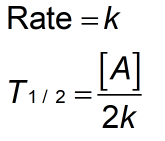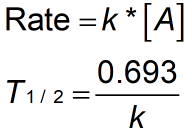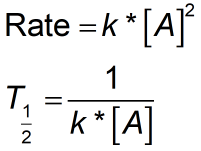Request a Tool

Rate Constant Calculator

Ratio or proportionality of the rate of reaction to the concentrations of the reactants

Reactin Rate
0
Half Life (T1/2)
0

Reaction Rate
0
Half Life(T1/2)
0

Reaction Rate
0
Half Life(T1/2)
0

Formula• k = Reaction constant
• [A] = Concentration
• = Half life

Defination / Uses

A rate constant is a term used in chemistry to define the ratio or proportionality of the rate of reaction to the concentrations of the reactants.

Formula• k = Reaction constant
• [A] = Concentration
• = Half life

Defination / Uses

A rate constant is a term used in chemistry to define the ratio or proportionality of the rate of reaction to the concentrations of the reactants.

Formula• k = Reaction constant
• [A] = Concentration
• = Half life

Defination / Uses

A rate constant is a term used in chemistry to define the ratio or proportionality of the rate of reaction to the concentrations of the reactants.

The Arrhenius equation may also be used to describe the rate constant:
k = Ae-Ea/RT
Here, A is the particle rate of the reaction constant, Ea is the maximum kinetic energy, R is the universal gas constant, and T is the absolute temperature. The Arrhenius equation shows that temperature is the most important element in determining the rate of a chemical reaction. The rate constant should, in theory, account for all of the variables that effect reaction rate. the unit of rate constant is depend on order of reaction. units of rate contact are mol1−(m+n)·L(m+n)−1·s−1.

Zero

The rate constant for a zero order reaction is measured in molar per second (M/s) or mole per litre per second (molL1s1).

First

The rate constant for a first-order reaction can be determined in per second of s-1.

Second

The rate constant for a second order reaction is measured in litres per mole per second (Lmol1s1) or milliseconds per second (M1s1). Use the upper given formula for manual calculations. No sign-up, registration OR captcha is required to use this tool.